Importance: Medium ✭✭
 Subject: Geometry
 Keywords: geometric complete graph, colouring
 Posted by: David Wood on: October 19th, 2009
Question   What is the minimum number of colours such that every complete geometric graph onvertices has an edge colouring such that:
\item[Variant A] crossing edges get distinct colours, \item[Variant B] disjoint edges get distinct colours, \item[Variant C] non-disjoint edges get distinct colours, \item[Variant D] non-crossing edges get distinct colours.

Letbe a set ofpoints in the plane with no three collinear. Draw a straight line-segment between each pair of points in. We obtain the complete geometric graph with vertex set, denoted by.

Two edges inare either:

\item adjacent if they have a vertex in common, \item crossing if they intersect at a point in the interior of both edges. \item disjoint if they do not intersect.

Let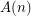,,andbe the minimum number of colours for the four variants.

Variant A: Here each colour class is a plane subgraph. Since there are point sets for which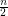edges are pairwise crossing,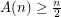. For an upper bound, say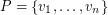. Colour each edge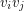withby colour. Each colour class is a non-crossing star. So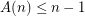. Bose et al [BHRW] improved this upper bound to.

Conjecture.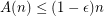for some.

Variant B: Here edges receiving the same colour must intersect. So each colour class is a geometric thrackle. Since there are point sets for whichedges are pairwise disjoint,. The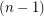-colouring given in Variant A also works here. So.

Conjecture.for some.

Variant C: Here each colour class is a plane matching. So each colour class has at mostedges, and thus at leastcolours are always needed. Thus. Araujo [ADHNU] proved an upper bound of.

Conjecture.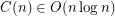.

Strong Conjecture..

Variant D: (This variant was recently mentioned in [Mat].) Here edges receiving the same colour must cross. Each colour class is called a crossing family [ADHNU]. Every edge in any triangulation ofrequires its own colour. So if the convex hull ofhas only three points, then at least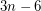colours are needed. Thus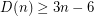.

Conjecture. A super-linear number of colours are always needed; i.e.,as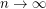.

A better lower bound is obtained by takingin convex position. Then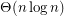is the minimum number of colours [KK]. I am not aware of any non-trivial upper bound for arbitrary point sets.

Bibliography

[ADHNU] G. Araujo, A. Dumitrescu, F. Hurtado, M. Noy, J. Urrutia, On the chromatic number of some geometric type Kneser graphs, Computational Geometry: Theory & Applications 32(1):59–69, 2005 MathSciNet

[BHRW] Prosenjit Bose, Ferran Hurtado, Eduardo Rivera-Campo, David R. Wood. Partitions of complete geometric graphs into plane trees, Computational Geometry: Theory & Applications 34(2):116-125, 2006. MathSciNet

[AEGKKPS] B. Aronov, P. Erdos, W. Goddard, D.J. Kleitman, M. Klugerman, J. Pach, L.J. Schulman, Crossing families, Combinatorica 14(2):127–134, 1994. MathSciNet

[KK] Alexandr Kostochka and Jan Kratochvil. Covering and coloring polygon-circle graphs, Discrete Math. 163(1--3):299--305, 1997. MathSciNet

[Mat] Jiří Matoušek. Blocking visibility for points in general position. Discrete Comput. Geom. 42(2):219--223, 2009. MathSciNet

* indicates original appearance(s) of problem.